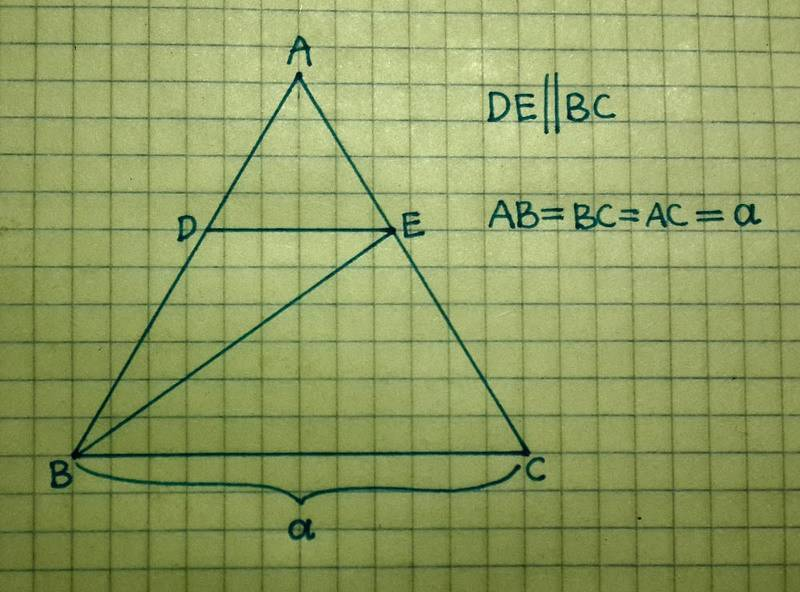# Maximum area of a triangle inscribed in another triangle?

Daveigh

## Homework Statement

[/B]
Hello!

I have this question which I don't quite know how to solve...

ABC is an equilateral triangle - the length of its sides equal to (a).
DE is parallel to BC

1. What length should DE be to achieve the largest possible area of triangle BDE?
2. What length should DE be to achieve the smallest possible perimeter of triangle BDE?

How should this be done (step-by-step, my knowledge of math is quite basic)?The attempt at a solution

I tried to solve the first question and got that DE should be (a/2) to make the maximum area of BDE. (I let DE = (a-a/x) and BD = (a/x), I used a formula to calculate area of a triangle using 2 sides and the angle between (120°) and then I derived the area expression...
But I think that what I was attempting is very messy, and I am unsure if the way I did it is correct.

Also, I didn't manage to solve the second question about the perimeter.
If anyone could explain and show the correct answer for comparison, I would be very grateful.

Mentor
I tried to solve the first question and got that DE should be (a/2) to make the maximum area of BDE. (I let DE = (a-a/x) and BD = (a/x), I used a formula to calculate area of a triangle using 2 sides and the angle between (120°) and then I derived the area expression...
But I think that what I was attempting is very messy, and I am unsure if the way I did it is correct.
The method looks fine and the answer is correct, that looks good.
It is easier if you use the formula A=1/2 (DE)*(height) because then you avoid dealing with odd angles.

Also, I didn't manage to solve the second question about the perimeter.
What did you get as perimeter?

Daveigh
I tried making an expression for the perimeter but when I differentiated it it was so large and I didn't know what to do next.
(a/x) + (a-a/x) + sqrt((a/x)^2+(a-a/x)^2-2*(a/x)*(a-a/x)*cos120°)
I couldn't get rid of 'a' to find 'x'...

Homework Helper
I tried making an expression for the perimeter but when I differentiated it it was so large and I didn't know what to do next.
(a/x) + (a-a/x) + sqrt((a/x)^2+(a-a/x)^2-2*(a/x)*(a-a/x)*cos120°)
I couldn't get rid of 'a' to find 'x'...

You can factor out a from the expression of the perimeter. Note that a/x + (a-a/x) = a.
Expand the parentheses under the square root and simplify before taking the derivative.

Last edited:
Mentor
There are two other tricks that can help:
The minimum of f(x) is also a minimum of f(x)+b with some constant b.
The minimum of f(x) is also a minimum of f2(x). The minimum of f2(x), if not zero, is a minimum OR maximum of f(x) depending on the sign of f(x).

But there is an even better approach: ehild's hint gives you the sum of two sides of the triangle, and it is constant. You just have to minimize the length of a specific side (which one?), and this can be done without calculations.